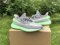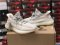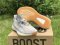USD
My Cart (0)

# 350 V2 original explosive, corporate level Item NO: 1436339

US\$ 179.99
Colorsize
US5=UK3 1/2=FR36=JP220=CHN220 US5 1/2=UK4=FR36 2/3=JP225=CHN225 US6=UK4 1/2=FR37 1/3=JP230=CHN230 US6 1/2=UK5=FR38=JP235=CHN235 US7=UK5 1/2=FR38 2/3=JP240=CHN235 US7 1/2=UK6=FR39 1/3=JP245=CHN240 US7=UK6 1/2=FR40=JP250=CHN245 US7 1/2=UK7=FR40 2/3=JP255=CHN250 US8=UK7 1/2=FR41 1/3=JP260=CHN255 US8 1/2=UK8=FR42=JP265=CHN260 US9=UK8 1/2=FR42 2/3=JP270=CHN265 US9 1/2=UK9=FR43 1/3=JP275=CHN265 US10=UK9 1/2=FR44=JP280=CHN270 US10 1/2=UK10=FR44 2/3=JP285=CHN275 US11=UK10 1/2=FR45 1/3=JP290=CHN280 US12=UK11 1/2=FR46 2/3=JP300=CHN290
 Customized Requirements
Quantity
Stock ( 4752 )